# Identifying and Working with Functions: Tutoring Solution Chapter Exam

Exam Instructions:

Choose your answers to the questions and click 'Next' to see the next set of questions. You can skip questions if you would like and come back to them later with the yellow "Go To First Skipped Question" button. When you have completed the practice exam, a green submit button will appear. Click it to see your results. Good luck!

Answered 0 of 30

### Page 2

#### Question 9 9. In the figure shown below, the graph of the green function is a translation of the graph of the red function. If the expression for the red function is g(x) = r(x), which of the following is the equation of the green function?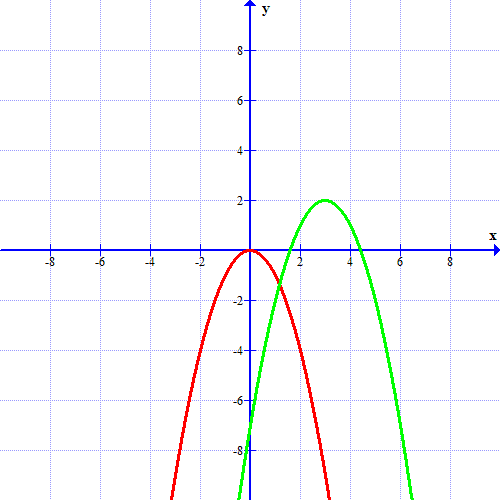### Page 3

#### Question 13 13. Evaluate f(f(x)). [Yes, it says f(f(x)]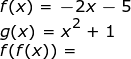#### Question 15 15. The revenue of a company is represented by R(x) and their costs are represented by C(x). What is the profit function R(x) - C(x)?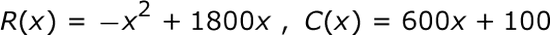### Page 4

#### Question 16 16. If the graph of f(x) is shown below, which choice represents the graph of f(x+1)+2 ?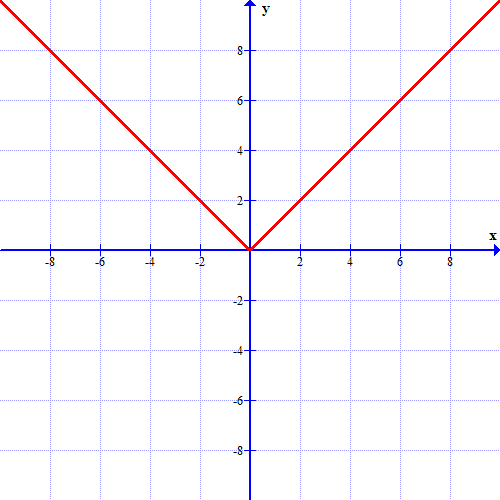#### Question 19 19. Evaluate.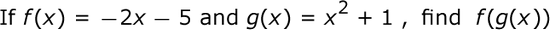#### Question 20 20. What is the range of the function represented by the graph below?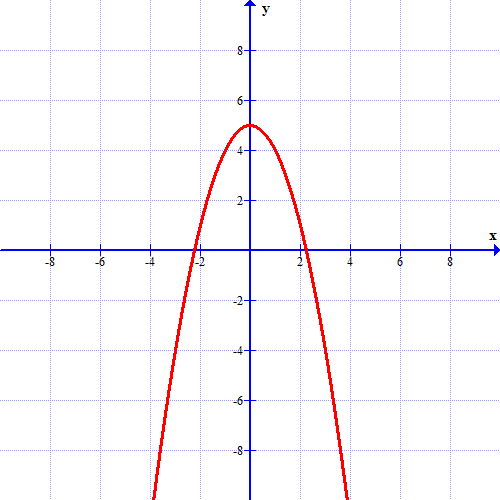### Page 5

#### Question 21 21. Which kind of transformation would change the red function below into the green one?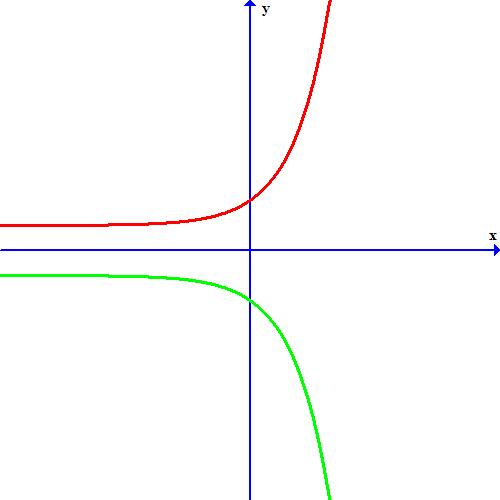#### Question 24 24. Using the function below, evaluate g(7).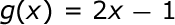### Page 6

#### Question 30 30. Find the domain of f(x)/g(x), if: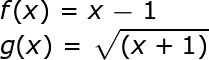#### Identifying and Working with Functions: Tutoring Solution Chapter Exam Instructions

Choose your answers to the questions and click 'Next' to see the next set of questions. You can skip questions if you would like and come back to them later with the yellow "Go To First Skipped Question" button. When you have completed the practice exam, a green submit button will appear. Click it to see your results. Good luck!

Support